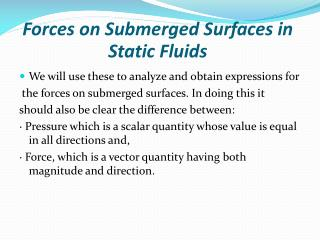DownloadDownload PresentationForces on Submerged Surfaces in Static Fluids

Forces on Submerged Surfaces in Static Fluids

Download PresentationForces on Submerged Surfaces in Static Fluids

- - - - - - - - - - - - - - - - - - - - - - - - - - - E N D - - - - - - - - - - - - - - - - - - - - - - - - - - -
Presentation Transcript

1. Forces on Submerged Surfaces in Static Fluids • We will use these to analyze and obtain expressions for the forces on submerged surfaces. In doing this it should also be clear the difference between: · Pressure which is a scalar quantity whose value is equal in all directions and, · Force, which is a vector quantity having both magnitude and direction.

2. The total area is made up of many elemental areas. • The force on each elemental area is always normal to the surface but, in general, each force is of different magnitude as the pressure usually varies. R = p1δA1 + p2δA2 + … = Σ pδA • If the surface is a plane the force can be represented by one single resultant force, acting at right-angles to the plane through the centre of pressure.

3. Horizontal submerged plane For a horizontal plane submerged in a liquid (or a plane experiencing uniform pressure over its surface), the pressure, p, will be equal at all points of the surface. Thus the resultant force will be given by R = pressure x plane area R = P x A

4. Curved submerged surface • If the surface is curved, each elemental force will be a different magnitude and in different direction but still normal to the surface of that element. • The resultant force can be found by resolving all forces into orthogonal co-ordinate directions to obtain its magnitude and direction. This will always be less than the sum of the individual forces, ΣpδA.

5. This plane surface is totally submerged in a liquid of density ρand inclined at an angle of θto the horizontal. Taking pressure as zero at the surface and measuring down from the surface, the pressure on an element δA , submerged a distance z, is given by p = ρ g z and the force on this element; F = p δA = ρ g z δA The resultant force is then; R = ρg Σ z δA

6. The term ΣzδA is known as the 1st Moment of Area of the plane PQ about the free surface. It is equal to A ž i.e. R = ρ g A ž Where ž is measured vertically, it also can be expressed as R = γŷ sinθ A Where ŷ is measured along the body (see the figure above). Both refer to the point G, the center of gravity of the body.

7. The calculated resultant force acts at a point known as the center of pressure, Yp Where Io is the moment of inertia of the plane. * A table for shapes’ area, center of gravity and moment of inertia will be distributed.

8. Example 1: A vertical trapezoidal gate with its upper edge located 5 m below free surface of water as shown. Determine the total pressure force and the center of pressure on the gate. 3 m 5 m 2 m 1 m

9. Solution: R = γ ž A R = 228900 N Io = 1.22 m4 ŷ = 5.83 m A = 4 m2 Yp = 5.88 m

10. Example 2: An inverted semicircle gate is installed at 45˚. The top of the gate is 5 m below the water surface. Determine the total pressure and center of pressure on the gate. ŷ 5 m R Yp 4 m

11. Solution: R = γŷ sinθ A A = ½ ( π/4 x 42) = 6.28 m2 ŷ = 5 sec 45˚ + (4 x 4)/3π = 8.77 m R = 382044 N Io = 25.13 m2 Yp = 9.23 m